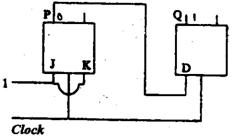Related Articles
GATE | GATE-CS-2000 | Question 35
• Last Updated : 19 Nov, 2018

The following arrangement of master-slave flip flops has the initial state of P, Q as 0, 1 (respectively). After three clock cycles the output state P, Q is (re­spectively),

``

(A) 1, 0
(B) 1, 1
(C) 0, 0
(D) 0,1

Explanation: Given P = 0 ,  J = 1 and k = 1

• JK flipflop toggles the input in ‘11’ state. Therefore, output of first flipflop at P is ‘1’.
• Initial value of P is input for D flipflop. So, D = 0 .Therefore, output of second flipflop at Q is ‘0’.

Thus, option (A) is the answer.

Please comment below if you find anything wrong in the above post.My Personal Notes arrow_drop_up
Recommended Articles
Page :